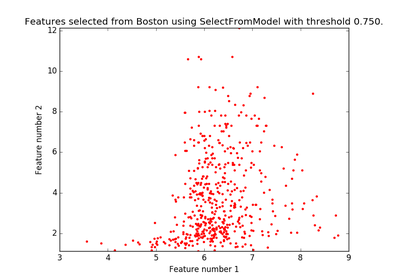# `sklearn.feature_selection`.SelectFromModel¶

class `sklearn.feature_selection.``SelectFromModel`(estimator, threshold=None, prefit=False)[source]

Meta-transformer for selecting features based on importance weights.

New in version 0.17.

Parameters: estimator : object The base estimator from which the transformer is built. This can be both a fitted (if `prefit` is set to True) or a non-fitted estimator. threshold : string, float, optional default None The threshold value to use for feature selection. Features whose importance is greater or equal are kept while the others are discarded. If “median” (resp. “mean”), then the `threshold` value is the median (resp. the mean) of the feature importances. A scaling factor (e.g., “1.25*mean”) may also be used. If None and if the estimator has a parameter penalty set to l1, either explicitly or implicitly (e.g, Lasso), the threshold used is 1e-5. Otherwise, “mean” is used by default. prefit : bool, default False Whether a prefit model is expected to be passed into the constructor directly or not. If True, `transform` must be called directly and SelectFromModel cannot be used with `cross_val_score`, `GridSearchCV` and similar utilities that clone the estimator. Otherwise train the model using `fit` and then `transform` to do feature selection. `estimator_`: an estimator : The base estimator from which the transformer is built. This is stored only when a non-fitted estimator is passed to the `SelectFromModel`, i.e when prefit is False. `threshold_`: float : The threshold value used for feature selection.

Methods

 `fit`(X[, y]) Fit the SelectFromModel meta-transformer. `fit_transform`(X[, y]) Fit to data, then transform it. `get_params`([deep]) Get parameters for this estimator. `get_support`([indices]) Get a mask, or integer index, of the features selected `inverse_transform`(X) Reverse the transformation operation `partial_fit`(X[, y]) Fit the SelectFromModel meta-transformer only once. `set_params`(\*\*params) Set the parameters of this estimator. `transform`(X) Reduce X to the selected features.
`__init__`(estimator, threshold=None, prefit=False)[source]
`fit`(X, y=None, **fit_params)[source]

Fit the SelectFromModel meta-transformer.

Parameters: X : array-like of shape (n_samples, n_features) The training input samples. y : array-like, shape (n_samples,) The target values (integers that correspond to classes in classification, real numbers in regression). **fit_params : Other estimator specific parameters self : object Returns self.
`fit_transform`(X, y=None, **fit_params)[source]

Fit to data, then transform it.

Fits transformer to X and y with optional parameters fit_params and returns a transformed version of X.

Parameters: X : numpy array of shape [n_samples, n_features] Training set. y : numpy array of shape [n_samples] Target values. X_new : numpy array of shape [n_samples, n_features_new] Transformed array.
`get_params`(deep=True)[source]

Get parameters for this estimator.

Parameters: deep : boolean, optional If True, will return the parameters for this estimator and contained subobjects that are estimators. params : mapping of string to any Parameter names mapped to their values.
`get_support`(indices=False)[source]

Get a mask, or integer index, of the features selected

Parameters: indices : boolean (default False) If True, the return value will be an array of integers, rather than a boolean mask. support : array An index that selects the retained features from a feature vector. If indices is False, this is a boolean array of shape [# input features], in which an element is True iff its corresponding feature is selected for retention. If indices is True, this is an integer array of shape [# output features] whose values are indices into the input feature vector.
`inverse_transform`(X)[source]

Reverse the transformation operation

Parameters: X : array of shape [n_samples, n_selected_features] The input samples. X_r : array of shape [n_samples, n_original_features] X with columns of zeros inserted where features would have been removed by transform.
`partial_fit`(X, y=None, **fit_params)[source]

Fit the SelectFromModel meta-transformer only once.

Parameters: X : array-like of shape (n_samples, n_features) The training input samples. y : array-like, shape (n_samples,) The target values (integers that correspond to classes in classification, real numbers in regression). **fit_params : Other estimator specific parameters self : object Returns self.
`set_params`(**params)[source]

Set the parameters of this estimator.

The method works on simple estimators as well as on nested objects (such as pipelines). The latter have parameters of the form `<component>__<parameter>` so that it’s possible to update each component of a nested object.

Returns: self :
`transform`(X)[source]

Reduce X to the selected features.

Parameters: X : array of shape [n_samples, n_features] The input samples. X_r : array of shape [n_samples, n_selected_features] The input samples with only the selected features.

## Examples using `sklearn.feature_selection.SelectFromModel`¶Feature selection using SelectFromModel and LassoCV